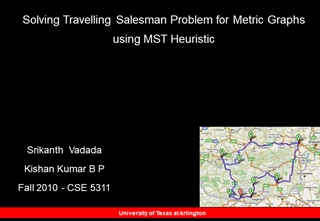# Platform as a Service - University of Texas at Arlington

0

#### Presentation Transcript

• 1. Srikanth Vadada Kishan Kumar B P Fall 2010 - CSE 5311 Solving Travelling Salesman Problem for Metric Graphs using MST Heuristic
• 2.Presentation Outline TSP Problem Overview TSP Approximation Algorithms Project Modules Project Demo
• 3.TSP Problem Overview TSP is a Graph Problem Where a salesman wishes to make a tour ,or Hamiltonian Cycle Visiting each city exactly once and Finishing at the city he starts from With the Lowest Path Cost TSP is a NP Complete Problem Optimum Solution can be from a Brute force approach Metric TSP Complete Graph TSP that satisfies the triangle inequality Approximation Algorithms for Metric TSP 2 - approximation algorithm 1.5 - approximation algorithm (Christofides Algorithm) Both algorithms use MST as the basic step
• 4.2 Approximation Algorithm Approximation Algorithm 1 1. Construct Minimum Spanning Tree 2. Creating a Cycle (MST Euler Tour) 3. Remove Redundant Visits Using Triangle Inequality 4. Wt (Approx) <= 2 * Wt(OPT) S M S E S B C B S A S Euler Path S M E B C A S TSP Cost - 64 M A B C S E M - Opt TSP Cost - 54
• 5.1.5 Approximation (Christofides Algorithm) Approximation Algorithm 2 1. Construct Minimum Spanning Tree 2. Take G restricted to odd degree vertices in MST as Subgraph G* Minimum Wt Matching M* on G* H = Union M* with MST Eulerian Tour of H Use Triangle Inequality to get Sol. 7. Wt (Approx) <= 1.5 * Wt(OPT) M A B C S E M - Opt TSP Cost - 54
• 6.Project Modules Functional Logic Kruskal’s Algorithm for MST (For 2 & 1.5 approx) Optimal TSP – Brute Force Method (For 2 & 1.5 approx) Finding Euler Cycle – Fleury’s Algorithm (For 2 & 1.5 approx) Minimum Weight Matching – Edmond’s Algorithm (For 1.5 approx ) Finding Short Circuit Path (For 2 & 1.5 approx) GUI Form based Interface
• 7.GUI Design   Core Java for Business Logic & Visual Basic Forms for GUI
• 8.References   . http://www.cs.tufts.edu/comp/260/tspscribe-final.pdf   . http://roticv.rantx.com/book/Eulerianpathandcircuit.pdf   . http://www.austincc.edu/powens/+Topics/HTML/05-6/05-6.html . http://people.math.sfu.ca/~goddyn/Courseware/edmonds.pdf
• 9.A Q & Questions ?
• 10.Time Complexity for 2 Approximation Algorithm : MST of G, use Kruskal’s or Prim’s Algorithm - O(E log V ). Finding a closed eulerian trail - O( E) using Fleury’s Algorithm Overall time complexity for 2 Approximation Algorithm - O( E log V ) Time Complexity for 1.5 Approximation Algorithm : MST of G, use Kruskal’s or Prim’s Algorithm - O(E log V ). Edmond’s Blossom Shrinking Algorithm and runs in O( V 3 ) Finding a closed eulerian trail - O(E) using Fleury’s Algorithm Overall time complexity for 1.5 Approximation Algorithm - O( V 3 ) Extra Slides for Reference - 1
• 11.Proof for Approx 2 Algorithm : Illegal Tour = 2 MST Wt (Legal Tour) <= 2 MST Wt (Optimal Tour) >= MST Wt (Legal Tour) <= 2 Optimal Tour Extra Slides for Reference - 2
• 12.Proof for Christofides Algorithm : A - Length of the tour computed by the Christofides Heuristic OPT - Optimal tour MST - Minimum Spanning Tree MOM - Minimum odd-vertex matching. The graph T U M, and therefore any Euler tour of T U M, has total length MST +MOM. By the triangle inequality, taking a shortcut past a previously visited vertex can only shorten the tour. Thus A <= MST +MOM. By the triangle inequality, the optimal tour of the odd-degree vertices of T cannot be longer than OPT. Any cycle passing through of the odd vertices can be partitioned into two perfect matchings,by alternately coloring the edges of the cycle red and green. One of these two matchings has length at most OPT/2. On the other hand, both matchings have length at least MOM. Thus, MOM <= OPT/2. Finally, recall our earlier observation that MST <= OPT. Putting these three inequalities together, we conclude that A <= 1.5 OPT Extra Slides for Reference - 3
• 13.Fleury's Algorithm 1.  pick any vertex to start 2.  from that vertex pick an edge to traverse (see below for important rule) 3.  darken that edge, as a reminder that you can't traverse it again 4.  travel that edge, coming to the next vertex 5.  repeat 2-4 until all edges have been traversed, and you are back at the starting vertex Extra Slides for Reference - 4
• 14.D E M O